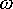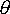QUESTION #202

# Could a circular space station simulate the Earth's gravity by spinning at a certain velocity? (I have seen something like this in A.C. Clarke's 2001)

Here is the long version:

Earth's gravity, simplified to what happens at the surface, says that the earth pulls at anyone with a force given by:

F = mg

If F is in Newtons, and m is in kilograms, g has the value of roughly 9.81 N/kg, and it is called the acceleration of gravity.

Now, if we set a cylindrical space station in rotational motion at a certain angular velocity, anything that moves with it will need a centripetal force to keep it rotating with the station. In other words, the 'floor' will have to push a person standing in it with a certain force, pretty much the same way the floor has to push every person up on earth. The magnitude of this force is given by:

F = m2r

Where m is the mass of the object,is the angular velocity of the space station and r is the radius of the space station.

Now, if we want to simulate Earth's gravity, we require that these two forces be equal.

So:

mg = m2r2r = g

Or

(2/T)2r = g

T = 2sqrt(r/g)

Where T is the period of rotation.

Given the size of the space station, it is then possible to figure out how fast it must be rotated. Let me give a few figures here.

For a radius of one mile, the space station would have to rotate with a period of about 80.5 seconds.

For a 10-mile radius space station, the period increases to about 4 minutes 15 seconds.

When you quadruple the radius, the period only doubles. So, it would seem that a rather fast paced rotation is required.

There is one caveat, however. Anything in motion inside the space station will be subject to what is called 'coriolis forces', which would not be experienced due to gravitational forces. The direction of the force is always perpendicular both to the axis of the space station, and the direction of motion, and its magnitude is

F = (2)/T * v * sin()

Where T is the period of the space station, v is the velocity of the body in motion, andis the angle between the axis and the direction of motion in the body. So, the faster the station rotates, the more the effect will be. So, one should try to make the station larger to minimize coriolis effects.
Answered by: Yasar Safkan, Ph.D. M.I.T., Software Engineer, Istanbul, Turkey